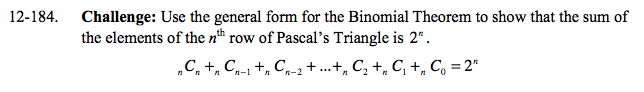### Home > A2C > Chapter 12 > Lesson 12.4.2 > Problem12-184

12-184.

Challenge: Use the general form for the Binomial Theorem to show that the sum of the elements of the nth row of Pascal's Triangle is 2n . Homework Help ✎ nCn + nCn−1 + nCn2 + …+ nC2 + nC1 + nC0 = 2nRefer to the Math Notes box in Lesson 12.4.2.

2n = (1 + 1)n so, substitute 1 for a and 1 for b.